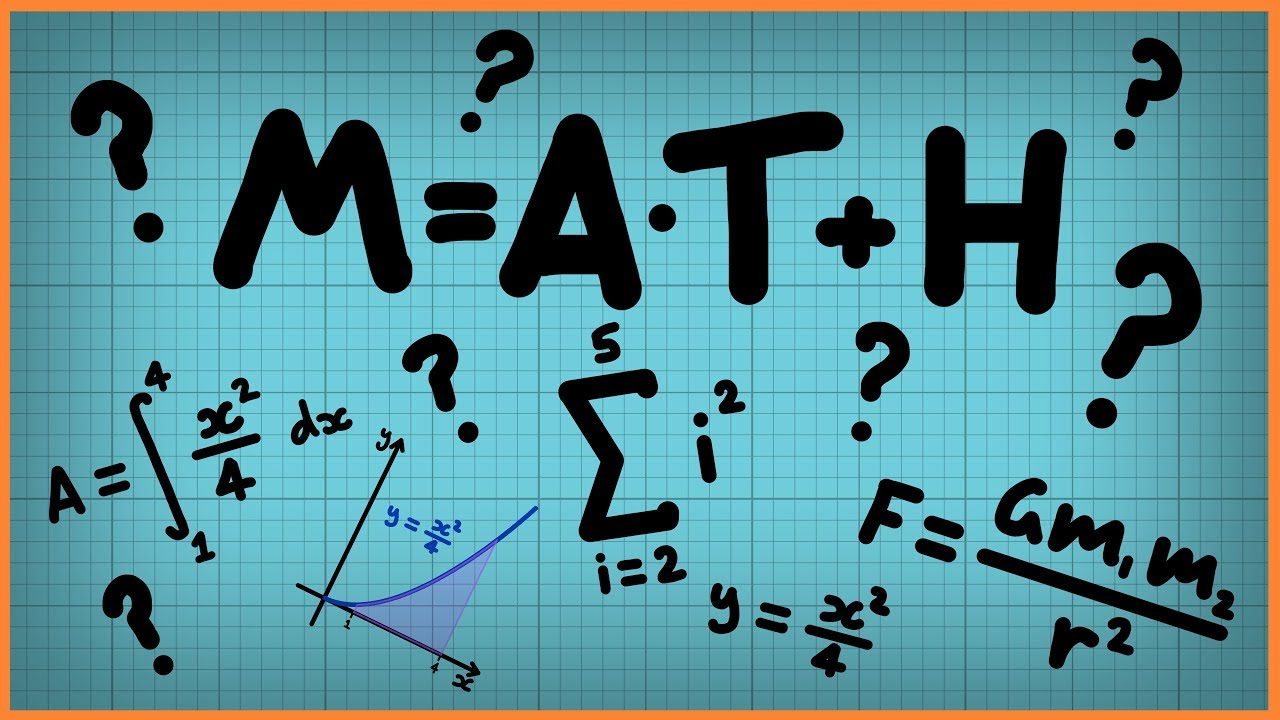Qalaxia Master Bot
0

I found an answer from byjus.com

Formula To Calculate Arc Length With Solved Examples

Example Questions Using the Formula for Arc Length. Question 1: Calculate the length of an arc if the radius of an arc is 8 cm and the central angle is 40°.

For more information, see Formula To Calculate Arc Length With Solved ExamplesGuru Nanak
0

Given that

Sun's diameter d = ?

Distance between the Sun and Earth D = 1.496 * 10^{11} m

Angular diameter of the sun \theta = 1920''

\theta = 1920 * 4.85 * 10^{-6} rad

\theta = 9.31 * 10^{-3} rad

Step 1: Develop a figure using the details provided.Step 2: Finding the diameter of the sun

From the figure:

AB is a diameter of the Earth

Assumption: AB is considering as a arc.

Arc length d = D \theta

d = 1.496 * 10^{11} * 9.31 * 10^{-3}

d = 1.39* 10^{9} m

Hence, diameter of the sun d = 1.39* 10^{9} m